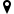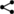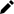Welcome guest
You're not logged in.
341 users online, thereof 0 logged in

## Proof: (related to "Basic Calculations Involving the Difference Operator")

By hypothesis, $D\subseteq\mathbb R$ is a subset of real numbers, $x, x+1\in D,\lambda\in\mathbb R,$ and $f,g:D\to\mathbb R$ are functions.

The statements follow immediately from the definition of the difference operator.

### Ad $(1)$

$$\begin{array}{rcl} \Delta (f\pm g)(x)&=&(f\pm g)(x+1)-(f\pm g)(x)\\ &=&f(x+1)\pm g(x+1)-(f(x)\pm g(x))\\ &=&(f(x+1)-f(x))\pm (g(x+1)-g(x))\\ &=&\Delta f(x)\pm \Delta g(x)\\ \end{array}$$

### Ad $(2)$

$$\begin{array}{rcl} \Delta (\lambda f)(x)&=&\lambda f(x+1)-\lambda f(x)\\ &=&\lambda(f(x+1)-f(x))\\ &=&\lambda \Delta f(x)\\ \end{array}$$

### Ad $(3)$

$$\begin{array}{rcl} \Delta (fg)(x)&=&(fg)(x+1)-(fg)(x)\\ &=&f(x+1)g(x+1)-f(x)g(x)\\ &=&f(x+1)g(x+1)-f(x+1)g(x)+f(x+1)g(x)-f(x)g(x)\\ &=&f(x+1)(g(x+1)-g(x))+g(x)(f(x+1)-f(x))\\ &=&f(x+1)\Delta g(x)+g(x)\Delta f(x)\\ \end{array}$$

### Ad $(4)$

• Let $g(x+1)g(x)\neq 0$ for all $$x\in D$$.
• It follows \begin{align} \Delta \left(\frac fg\right)(x)&=\frac{f(x+1)}{g(x+1)}-\frac{f(x)}{g(x)}\\ &=\frac{g(x)f(x+1)-f(x)g(x+1)}{g(x+1)g(x)}\\ &=\frac{g(x)f(x+1)-g(x)f(x)-(f(x)g(x+1)-f(x)g(x))}{g(x+1)g(x)}\\ &=\frac{g(x)\Delta f(x)-f(x)\Delta g(x)}{g(x+1)g(x)}\\ \end{align}
q.e.d|||| created: 2020-03-24 17:24:48 | modified: 2020-03-24 17:25:31 | by: | references: , ,